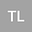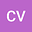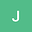Formulas copied from PDFs
•••• Tara Louis,
• Christine Verzella,
• j.goldsmith
Tara Louis
Author ProfileChristine Verzella
Author Profilej.goldsmith
Author Profile## Abstract

In this paper, we study the calibration to market call prices C^{mkt}(t;K) of a local volatility model enhanced with jumps. Instead of giving an exact calibration condition on the local volatility, we introduce an approximate process S_t^\epsilon satisfying a well-defined nonlinear McKean SDE driven by a Cox process, such that E[(S_t^\epsilon - K) _ ] converges to C^{mkt}(t;K) as \epsilon goes to 0 for all (t;K). This implies that the particle method, applied to the process S_t^\epsilon, which is used for the calibration of the local volatility, converges numerically. We illustrate the accuracy of our calibration algorithm with various numerical experiments. Finally, we extend this model by allowing jumps in the local volatility.